# Texas Go Math Grade 4 Lesson 10.3 Answer Key Model Division with Regrouping

Refer to our Texas Go Math Grade 4 Answer Key Pdf to score good marks in the exams. Test yourself by practicing the problems from Texas Go Math Grade 4 Lesson 10.3 Answer Key Model Division with Regrouping.

## Texas Go Math Grade 4 Lesson 10.3 Answer Key Model Division with Regrouping

Essential Question

How can you use base-ten blocks or on algorithm to model division with regrouping?

Investigate

Materials

• base-ten
• blocks

The librarian wants to share 54 books equally among 3 classes. How many books will she give to each class?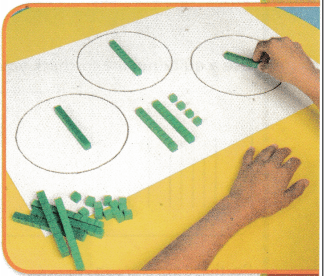A. Draw 3 circles to represent the classes. Then use base-ten blocks to model 54. Show 54 as 5 tens and 4 ones.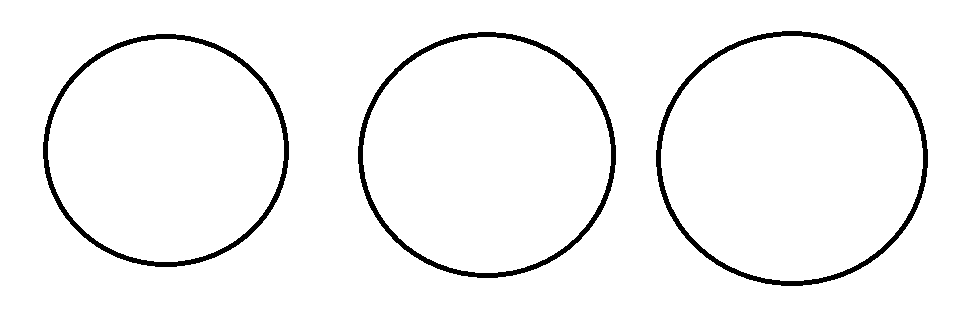B. Share the tens equally among the 3 groups.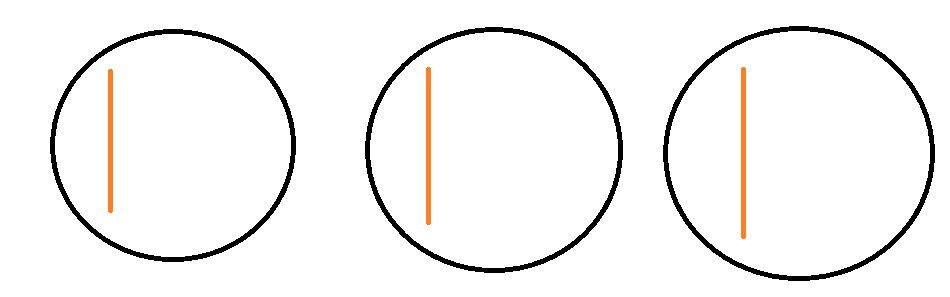C. If there are any tens left, regroup them as ones. Share the ones equally among the 3 groups.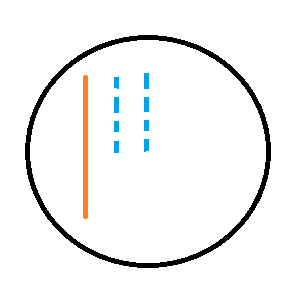D. There are __________ ten(s) and __________ one(s) in each group.

So, the librarian will give __________ books to each class.

Therefore, there are 1 tens and 8 ones in each group

So, the libraruan will give 18 books to each class.

Make Connections

Divide. 76 ÷ 3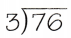Record each step.

STEP 1
Share the 7 tens equally among 3 groups.
There are __________ tens in each group.
__________ tens were used. There is __________ ten left over.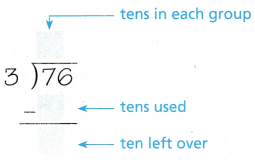Divisor is 3 and the Dividend is 76

We get 7 – 6 = 1

STEP 2
One ten cannot be shared among 3 groups without regrouping.
Regroup 1 ten.
There are now ___________ ones to share.Now, 7 – 6 = 1

Now, the remainder bcomes 16

STEP 3
Share the ones equally among 3 groups.
There are ___________ ones in each group.
___________ ones were shared. There is ___________ one left over.Now, 3 x 5 = 15

So, 16 – 15 = 1

Therefore, Quotient = 25

Remainder = 1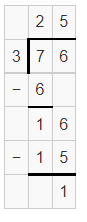Use a quick picture to check your work.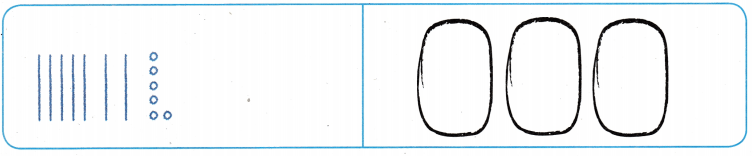So, there are 3 groups of ___________ and ___________ left over.So, there are 3 groups of 25 and1 left over.

Share and Show

Divide. Lise base-ten blocks.

Question 1.
48 ÷ 3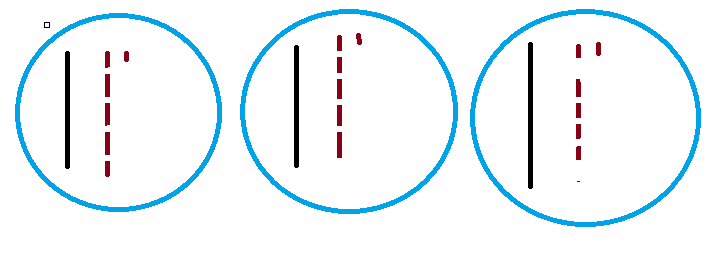Question 2.
84 ÷ 4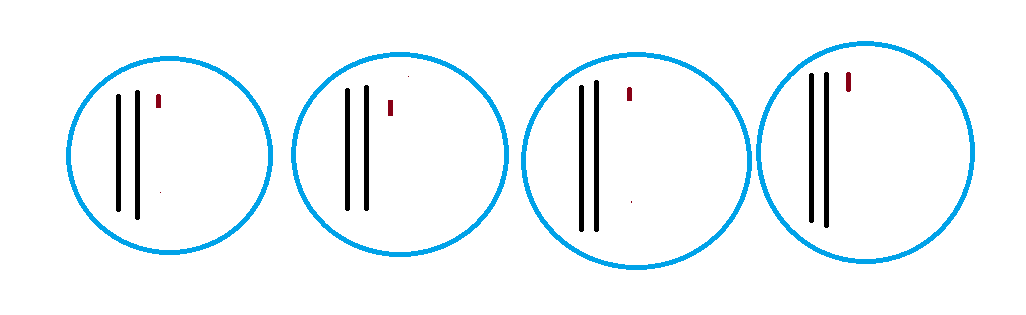Question 3.
72 ÷ 5Remainder :2

Divide. Record the steps. Draw quick pictures to check your work.

Question 4.
59 ÷ 2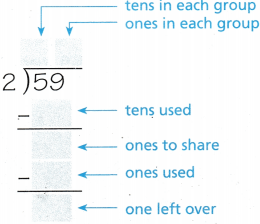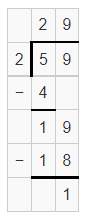Problem Solving

Sense or Nonsense?

Question 5.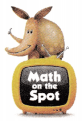H.O.T. Multi-Step Use Diagrams Angela and Zach drew quick pictures to find 68 ÷ 4. Whose quick picture makes sense? Whose quick picture is nonsense? Explain your reasoning.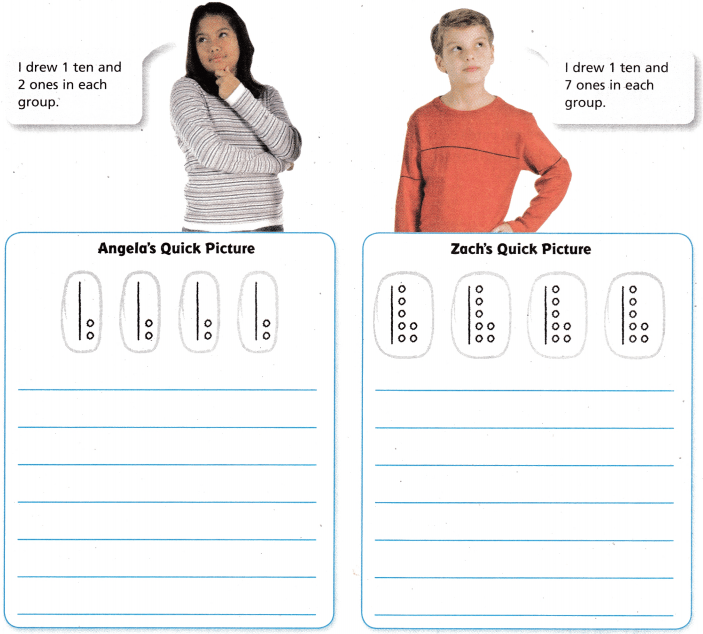68/4 = 17Zach’s quick picture is correct.

Angela’s quick picture is non sense.

Zach divided the tens and add the leftover tens as ones whereas Angela left the leftover tens. That is why Zach’s answer is accurate.

Question 6.
H.O.T. Analyze What did Angela forget to do after she shared the tens equally among the 4 groups?

Angela did not convert the leftover tens as ones that is why her answer is not correct. In ten-block model we have to convert the remaining tens in to ones and drew them equally in the correct place.

Question 7.
Use Tools Lisa has 51 small toys. She puts an equal number of toys in each of 3 bottles. How many toys does Lisa put in each bottle? Use base-ten blocks or a quick picture to solve.
(A) 10
(B) 14
(C) 17
(D) 18

Number of small toys =51

Given, She puts an equal number of toys in each of 3 bottles

Now, 51/3= 17Therefore, Lisa kept 17 toys in each bottle.

Question 8.
Jay shares 84 baseball cards equally among 6 friends. How many baseball cards does he give each friend?
(A) 13
(B) 20
(C) 1o
(D) 14

Number of baseball cards Jay have = 84

Number of friends = 6

Now, 84/6 = 14Question 9.
Multi-Step A grocer has 78 grape juice boxes. He puts an equal number of them on each of 3 shelves. The grocer has 96 apple juice boxes. he puts an equal number of them on each of 4 shelves. How many more grape juice boxes than apple juice boxes are on each shelf?
(A) 4
(B) 2
(C) 6
(D) 1

Number of grape juice boxes = 78

Number of shelves he kept = 3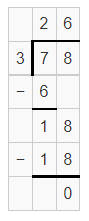78/3 = 26

Also, Number of apple juice boxes = 96

Number of shelves = 4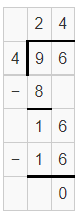96/4 = 24

Now, difference = 26 – 24 = 2

Therefore, the difference is 2 shelves.

TEXAS Test Prep

Question 10.
Parker rode his bike a total of 85 miles over five weeks. He rode the same number of miles each week. How far did he ride each week?
(A) 80 miles
(B) 11 miles
(C) 16 miles and 5 miles left over
(D) 17 miles

Number of weeks = 5

Number of miles Parker travelled = 85

Now, 85/5 =Therefore, he travelled 17 miles each week.

### Texas Go Math Grade 4 Lesson 10.3 Homework and Practice Answer Key

Divide. Use base-ten blocks.

Question 1.
68 ÷ 4 _________68/4 = 17

Question 2.
39 ÷ 3 _________39/3=13

Question 3.
75 ÷ 6 _________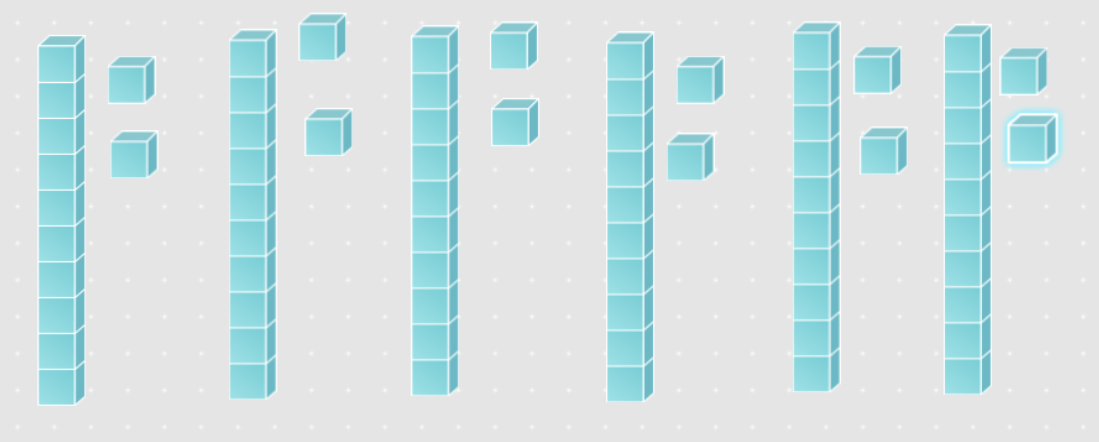75/6 = 12

Remainder : 5

Question 4.
84 ÷ 4 _________84/4 =21

Question 5.
96 ÷ 9 _________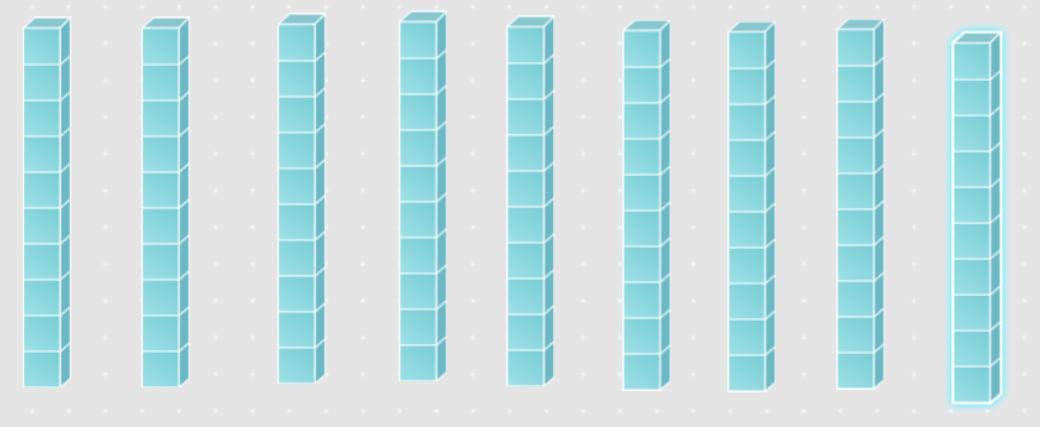96/9 =10

Remainder =6

Question 6.
60 ÷ 4 _________60/4 = 15

Divide. Record the steps. Draw quick pictures to check your work.

Question 7.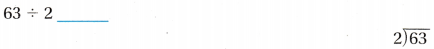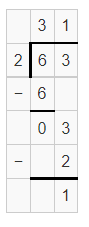Quotient : 31

Remainder :1

Question 8.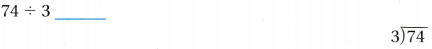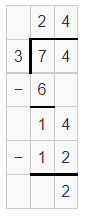Quotient : 24

Remainder :2

Problem Solving

Question 9.
There are 48 students ready to run relay races on field day. How many teams of 4 can be formed?

Number of students = 48

Number of teams should be formed = 4

Now, 48/4 =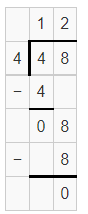therefore, 12 teams of 4 can be formed.

Question 10.
A total of 78 students will go on the Saturday field trip to the museum. Each van can carry 6 students. What is the least number of vans needed?

Number of students = 78

The number of students the van carries = 6

Which means, 78/6 =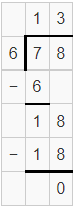Therefore, 13 vans are required.

Lesson Check

Question 11.
Laura picked 90 flowers and divided them equally into 6 vases. How many flowers are in each vase?
(A) 18
(B) 9
(C) 12
(D) 15

Total number of flowers = 90

Number of vases = 6

Now,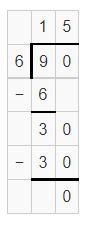Therefore, the number of flowers in each vase = 15

Question 12.
A jewelry store clerk has 72 rings to display. The clerk arranges the rings in 6 equal rows. How many rings are in each row?
(A) 12
(B) 14
(C) 10
(D) 13

The total number of rings = 72

Number of rows = 6

Now, 72/6

=Therefore, the are 12rings in each row.

Question 13.
Chris has collected 96 leaves for his nature hook. If he places 6 leaves on each page, how many pages will he use?
(A) 16
(B) 11
(C) 15
(D) 12

Number of collected leaves= 96

Number of leaves he kept in each page = 6

Now, 96/6 = 16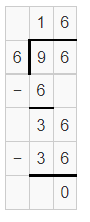Question 14.
At the plant store, there are 64 flowerpots divided equally onto 4 shelves. How many flowerpots are on each shelf?
(A) 12
(B) 14
(C) 16
(D) 21

Number of flower pots = 64

Number of shelves = 4

Now, 64/4 =Therefore, there are 16 flower pots in each shelf.

Question 15.
Multi-Step Mindy is preparing cookie boxes for gifts. She divides 36 chocolate chip cookies evenly into 6 boxes. Then she divided 54 sugar cookies evenly into the same 6 boxes. How many cookies are in each of Mindy’s boxes?
(A) 12
(B) 15
(C) 8
(D) 16

Number of chocolate chips = 36

Number of boxes = 6

Now,

36/6 =Also, Number of sugar cookies = 54

Now, 54/6 =Total number of cookies = 6 + 9 = 15

Question 16.
Multi-Step A gardener has 52 red rose bushes and 40 yellow rose bushes. He plants an equal number of each color in 4 gardens. How many more red rose bushes than yellow rose bushes are in each garden?
(A) 4
(B) 13
(C) 10
(D) 3

Number of red rose bushes = 52

Number of yellow rose bushes = 40

Total number = 52 +40

= 92

Number of gardens = 4

Now,

92/4 =Scroll to Top